Function Repository Resource:

# EulerLinePoints

Get the four main points of the Euler line of a triangle

Contributed by: Ed Pegg Jr
 ResourceFunction["EulerLinePoints"][{p1,p2,p3}] returns the circumcenter, centroid, nine-point center and orthocenter of the triangle defined by vertices p1,p2 and p3.

## Details

The circumcenter, centroid, nine-point center and orthocenter of a triangle all lie on the Euler line.
ResourceFunction["EulerLinePoints"][Triangle[{p1,p2,p3}]] is equivalent to ResourceFunction["EulerLinePoints"][{p1,p2,p3}].

## Examples

### Basic Examples (1)

Find the defining points for the Euler line of three triangle vertices:

 In:=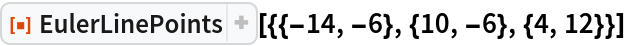Out=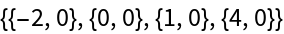### Scope (2)

Compute points on the Euler line of a 3D triangle:

 In:=Out=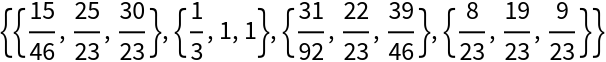Show the points and the triangle together:

 In:=Out=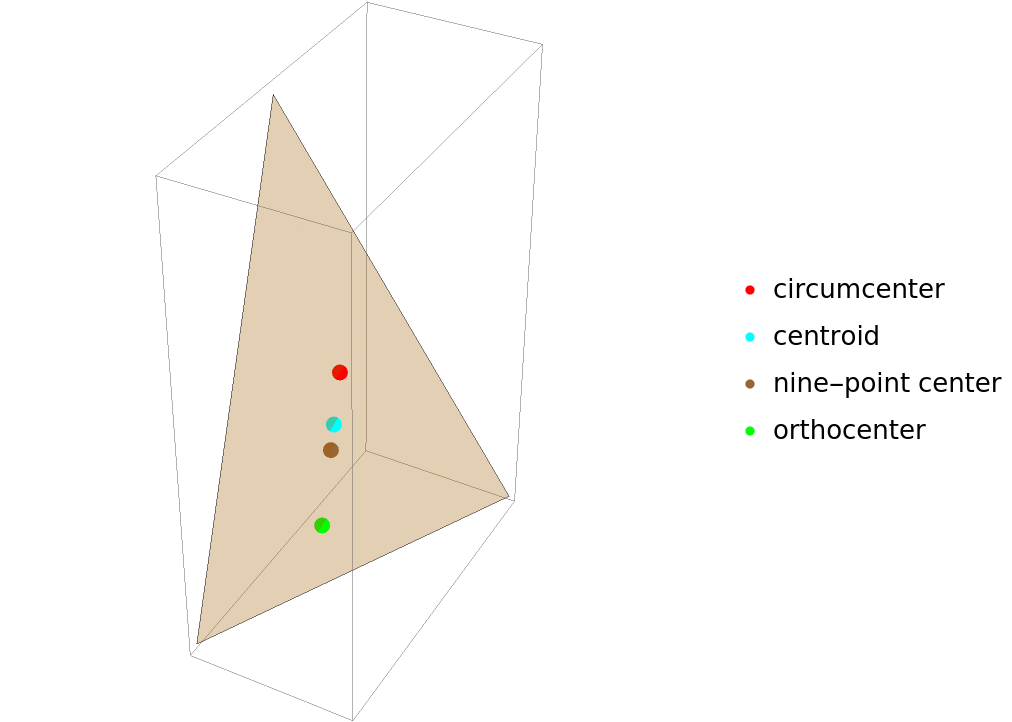### Neat Examples (1)

A graphic of a triangle with the Euler line (blue), circumcenter|circumcircle|perpendicular bisectors (red), centroid|medians (cyan), nine-point center|circle (brown) and orthocenter|altitudes (green):

 In:=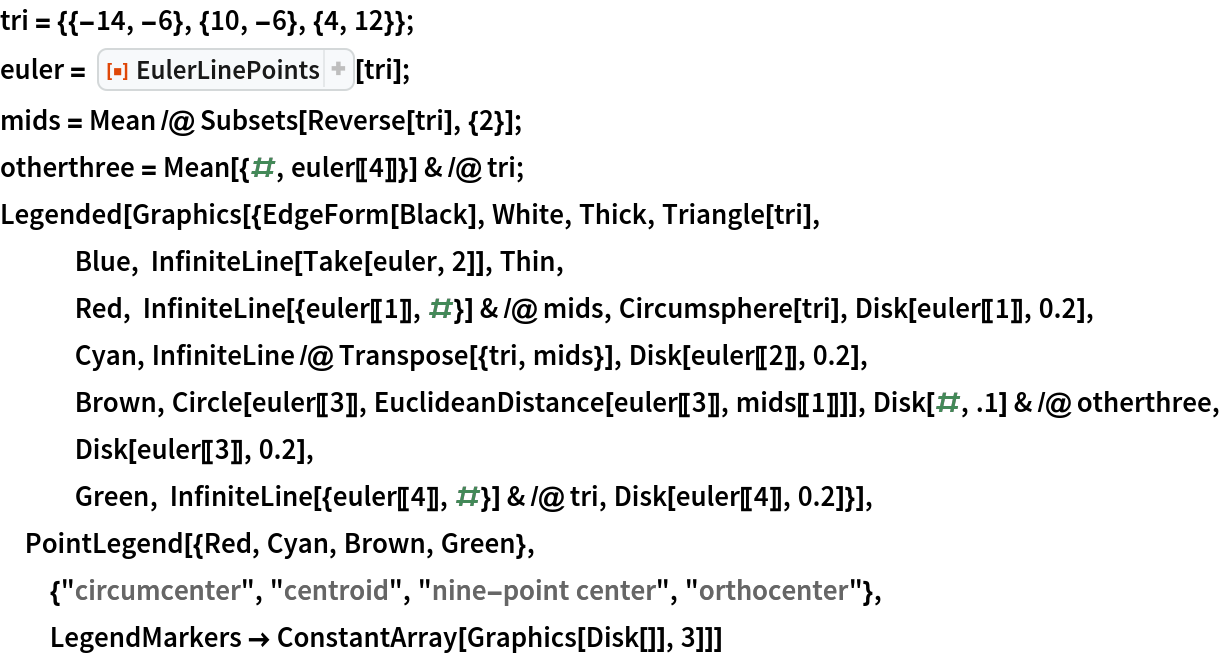Out=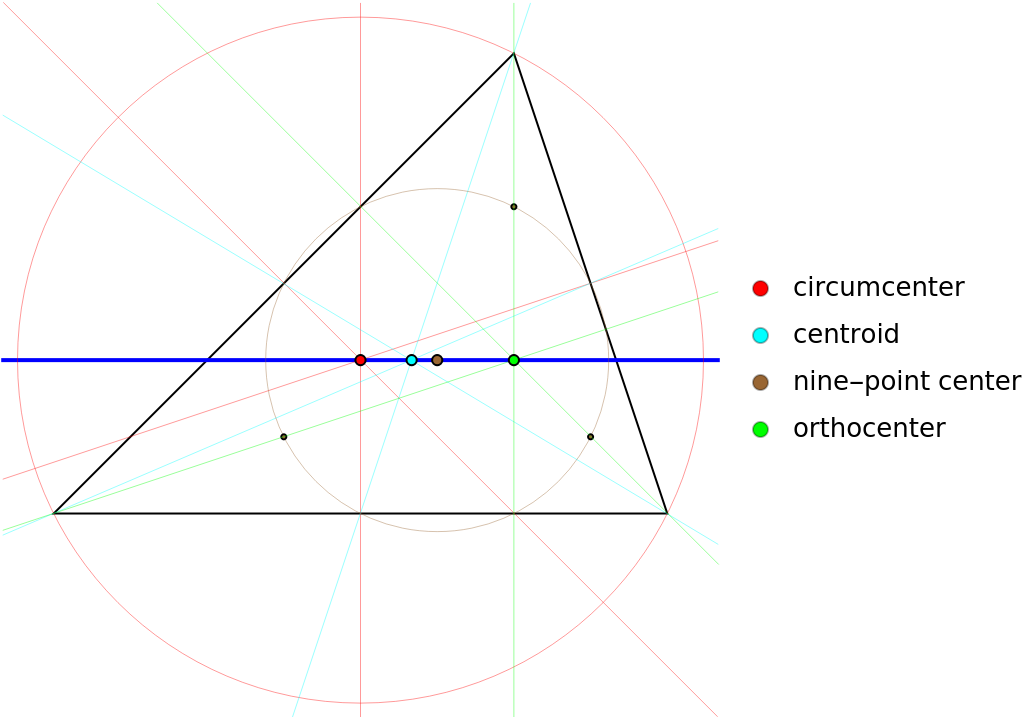## Version History

• 1.1.0 – 31 August 2021
• 1.0.0 – 17 October 2019